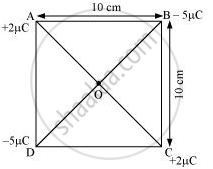# Four point charges qA = 2 μC, qB = −5 μC, qC = 2 μC, and qD = −5 μC are located at the corners of a square ABCD of side 10 cm. What is the force on a charge of 1 μC placed at the centre of the square? - Physics

Numerical

Four point charges q= 2 μC, q= −5 μC, qC = 2 μC, and qD = −5 μC are located at the corners of a square ABCD of side 10 cm. What is the force on a charge of 1 μC placed at the centre of the square?

#### Solution

The given figure shows a square of a side 10 cm with four charges placed at its corners. O is the centre of the square.Where,

(Sides) AB = BC = CD = AD = 10 cm

(Diagonals) AC = BD = 10sqrt2cm

AO = OC = DO = OB = 5sqrt2 cm

A charge of amount 1 μC is placed at point O.

Force of repulsion between charges placed at corner A and centre O is equal in magnitude but opposite in direction relative to the force of repulsion between the charges placed at corner C and centre O. Hence, they will cancel each other. Similarly, the force of attraction between charges placed at corner B and centre O is equal in magnitude but opposite in direction relative to the force of attraction between the charges placed at corner D and centre O. Hence, they will also cancel each other. Therefore, the net force caused by the four charges placed at the corner of the square on 1 μC charge at centre O is zero.

Concept: Coulomb’s Law - Force Between Two Point Charges
Is there an error in this question or solution?

#### APPEARS IN

NCERT Physics Part 1 and 2 Class 12
Chapter 1 Electric Charges and Fields
Exercise | Q 1.6 | Page 46
NCERT Class 12 Physics Textbook
Chapter 1 Electric Charge and Fields
Exercise | Q 6 | Page 46
Share